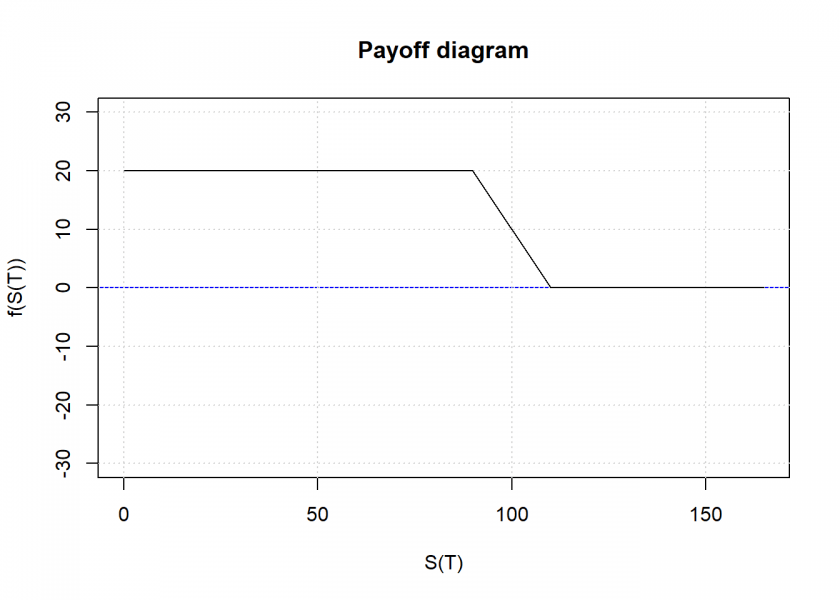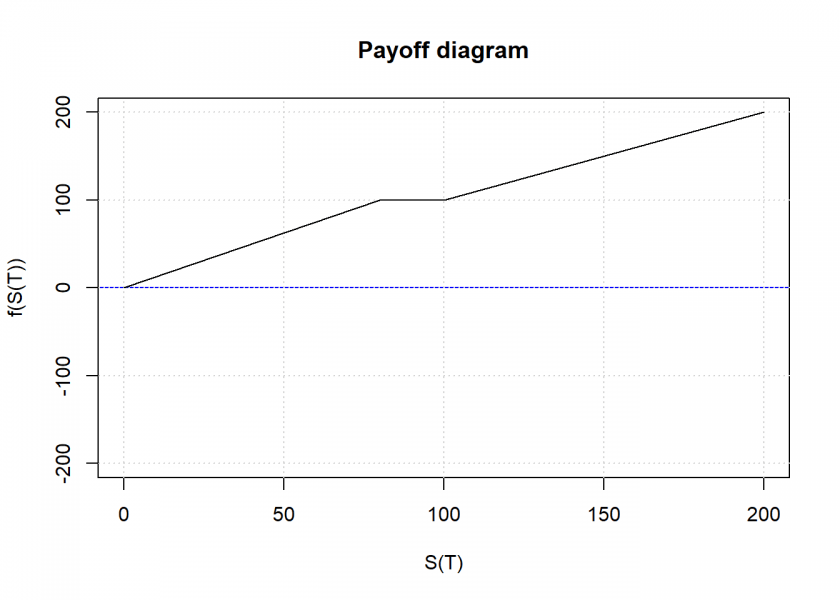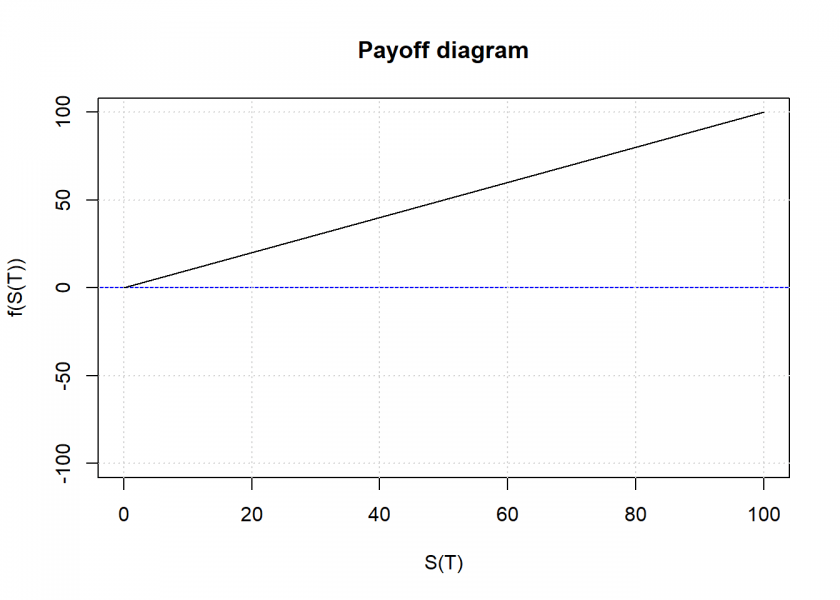Want to share your content on R-bloggers? click here if you have a blog, or here if you don't.In the area of option strategy trading, it has always been a dream of mine to have a universal tool that is able to replicate any payoff function statically by combining plain vanilla products like calls, puts, and zerobonds.

Many years ago there was such a tool online but it has long gone since and the domain is inactive. So, based on the old project paper from that website I decided to program it in R and make it available for free here!

The project paper the algorithm is based on and which is translated to R can be found here: Financial Engineering Tool: Replication Strategy and Algorithm. I will not explain the algorithm as such and how it works because this is done brilliantly in the paper. Also, I won’t get into any details concerning derivatives and structured products either. You can find tons of material on the web just by googling. So, without further ado let’s get started!

First, we need a way to define the payoff function: for each kink we provide two values, one for the underlying which goes from 0 to infinity and one for the payoff we want to replicate. We will use the names used in the paper for all the needed variables for clarity. Let us start by defining a plain vanilla call:

```payoff <- data.frame(pi = c(0, 100, 110, Inf), f_pi = c(0, 0, 10, Inf))
payoff
##    pi f_pi
## 1   0    0
## 2 100    0
## 3 110   10
## 4 Inf  Inf
```

The last value of the payoff must be either equal to the penultimate value (= payoff staying flat at the given value) or must be (minus) infinity for a linear continuation in the given direction. Next we want to plot this payoff:

```plot_payoff <- function(payoff, xtrpol = 1.5) {
k <- nrow(payoff) - 1
payoff_prt <- payoff
payoff_prt\$pi[k+1] <- payoff\$pi[k] * xtrpol
# linear extrapolation of last kink
slope <- diff(c(payoff\$f_pi[k-1], payoff\$f_pi[k])) / diff(c(payoff\$pi[k-1], payoff\$pi[k]))
payoff_prt\$f_pi[k+1] <- ifelse(payoff\$f_pi[k] == payoff\$f_pi[k+1], payoff\$f_pi[k+1], payoff\$f_pi[k] + payoff\$pi[k] * (xtrpol - 1) * slope)
plot(payoff_prt, ylim = c(-max(abs(payoff_prt\$f_pi) * xtrpol), max(abs(payoff_prt\$f_pi) * xtrpol)), main = "Payoff diagram", xlab = "S(T)", ylab = "f(S(T))", type = "l")
abline(h = 0, col = "blue")
grid()
lines(payoff_prt, type = "l")
invisible(payoff_prt)
}

plot_payoff(payoff)
```Now comes the actual replication. We need to functions for that: a helper function to calculate some parameters…

```calculate_params <- function(payoff) {
params <- payoff
k <- nrow(params) - 1

params\$s_f_pi <- ifelse(params\$f_pi < 0, -1, 1)
# linear extrapolation of last kink
slope <- diff(c(params\$f_pi[k-1], params\$f_pi[k])) / diff(c(params\$pi[k-1], params\$pi[k]))
f_pi_k <- ifelse(params\$f_pi[k] == params\$f_pi[k+1], params\$f_pi[k+1], slope)
params\$lambda <- c(diff(params\$f_pi) / diff(params\$pi), f_pi_k)
params\$s_lambda <- ifelse(params\$lambda < 0, -1, 1)
# consolidate
params[k, ] <- c(params[k, 1:3], params[(k+1), 4:5])
params <- params[1:k, ]
params
}
```

…and the main function with the replication algorithm:

```replicate_payoff <- function(payoff) {
params <- calculate_params(payoff)
suppressMessages(attach(params))
k <- nrow(params)

portfolios <- as.data.frame(matrix("", nrow = k, ncol = 6))
colnames(portfolios) <- c("zerobonds", "nominal", "calls", "call_strike", "puts", "put_strike")

# step 0 (initialization)
i <- 1
i_r <- 1
i_l <- 1

while (i <= k) {

# step 1 (leveling)
if (f_pi[i] != 0) {
portfolios[i, "zerobonds"] <- s_f_pi[i]
portfolios[i, "nominal"] <- abs(f_pi[i])
}

# step 2 (replication to the right)
while (i_r <= k) {
if (i_r == i) {
if (lambda[i] != 0) {
portfolios[i, "calls"] <- paste(portfolios[i, "calls"], lambda[i])
portfolios[i, "call_strike"] <- paste(portfolios[i, "call_strike"], pi[i])
}
i_r <- i_r + 1
next
}
if ((lambda[i_r] - lambda[i_r-1]) != 0) {
portfolios[i, "calls"] <- paste(portfolios[i, "calls"], (lambda[i_r] - lambda[i_r-1]))
portfolios[i, "call_strike"] <- paste(portfolios[i, "call_strike"], pi[i_r])
}
i_r <- i_r + 1
}

# step 3 (replication to the left)
while (i_l != 1) {
if (i_l == i) {
if (-lambda[i_l-1] != 0) {
portfolios[i, "puts"] <- paste(portfolios[i, "puts"], -lambda[i_l-1])
portfolios[i, "put_strike"] <- paste(portfolios[i, "put_strike"], pi[i_l])
}
} else {
if ((lambda[i_l] - lambda[i_l-1]) != 0) {
portfolios[i, "puts"] <- paste(portfolios[i, "puts"], (lambda[i_l] - lambda[i_l-1]))
portfolios[i, "put_strike"] <- paste(portfolios[i, "put_strike"], pi[i_l])
}
}
i_l <- i_l - 1
}

# step 4
i <- i + 1
i_r <- i
i_l <- i
}

# remove duplicate portfolios
portfolios <- unique(portfolios)
# renumber rows after removal
row.names(portfolios) <- 1:nrow(portfolios)
portfolios
}
```

Let us test our function for the plain vanilla call:

```replicate_payoff(payoff)
##   zerobonds nominal calls call_strike  puts put_strike
## 1                       1         100
## 2         1      10     1         110  -1 1    110 100
```

There are always several possibilities for replication. In this case, the first is just our call with a strike of 100. Another possibility is buying a zerobond with a nominal of 10, going long a call with strike 110 and simultaneously going short a put with strike 110 and long another put with strike 100.

Let us try a more complicated payoff, a classic bear spread (which is also the example given in the paper):

```payoff <- data.frame(pi = c(0, 90, 110, Inf), f_pi = c(20, 20, 0, 0))
payoff
##    pi f_pi
## 1   0   20
## 2  90   20
## 3 110    0
## 4 Inf    0

plot_payoff(payoff)
``````replicate_payoff(payoff)
##   zerobonds nominal calls call_strike  puts put_strike
## 1         1      20  -1 1      90 110
## 2                                      1 -1     110 90
```

Or for a so-called airbag note:

```payoff <- data.frame(pi = c(0, 80, 100, 200, Inf), f_pi = c(0, 100, 100, 200, Inf))
payoff
##    pi f_pi
## 1   0    0
## 2  80  100
## 3 100  100
## 4 200  200
## 5 Inf  Inf

plot_payoff(payoff, xtrpol = 1)
``````replicate_payoff(payoff)
##   zerobonds nominal         calls call_strike        puts  put_strike
## 1                    1.25 -1.25 1    0 80 100
## 2         1     100             1         100       -1.25          80
## 3         1     200             1         200  -1 1 -1.25  200 100 80
```

As a final example: how to replicate the underlying itself? Let’s see:

```payoff <- data.frame(pi = c(0, 100, Inf), f_pi = c(0, 100, Inf))
payoff
##    pi f_pi
## 1   0    0
## 2 100  100
## 3 Inf  Inf

plot_payoff(payoff, 1)
``````replicate_payoff(payoff)
##   zerobonds nominal calls call_strike puts put_strike
## 1                       1           0
## 2         1     100     1         100   -1        100
```

The first solution correctly gives us what is called a zero-strike call, i.e. a call with the strike of zero!

I hope you find this helpful! If you have any questions or comments, please leave them below.

I am even thinking that it might be worthwhile to turn this into a package and put it on CRAN, yet I don’t have the time to do that at the moment… if you are interested in cooperating on that please leave a note in the comments too. Thank you!